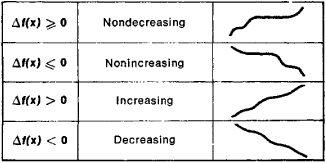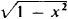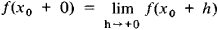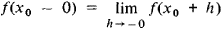# Monotonic Function

(redirected from Decreasing operator)

## monotonic function

[¦män·ə¦tän·ik ′fəŋk·shən]
(mathematics)
McGraw-Hill Dictionary of Scientific & Technical Terms, 6E, Copyright © 2003 by The McGraw-Hill Companies, Inc.
The following article is from The Great Soviet Encyclopedia (1979). It might be outdated or ideologically biased.

## Monotonic Function

(or monotone function), a function whose increments Δf(x) = f(x′) − f(x) do not change sign when Δx = x′ − x > 0; that is, the increments are either always nonnegative or always nonpositive. Somewhat inaccurately, a monotonic function can be defined as a function that always varies in the same direction. Different types of monotonic functions are represented in Figure 1. For example, the function y = x3 is an increasing function. If a function f(x) has a derivative f′(x) that is nonnegative at every point and that vanishes only at a finite number of individual points, then f(x) is an increasing function. Similarly, if f′(x) ≤ 0 and vanishes only at a finite number of points, then f(x) is a decreasing function.Figure 1

A monotonicity condition can hold either for all x or for x on a given interval. In the latter case, the function is said to be monotonic on this interval. For example, the function y =increases on the interval [−1,0] and decreases on the interval [0, +1]. A monotonic function is one of the simplest classes of functions and is continually encountered in mathematical analysis and the theory of functions. If f(x) is a monotonic function, then the following limits exist for any X0:and